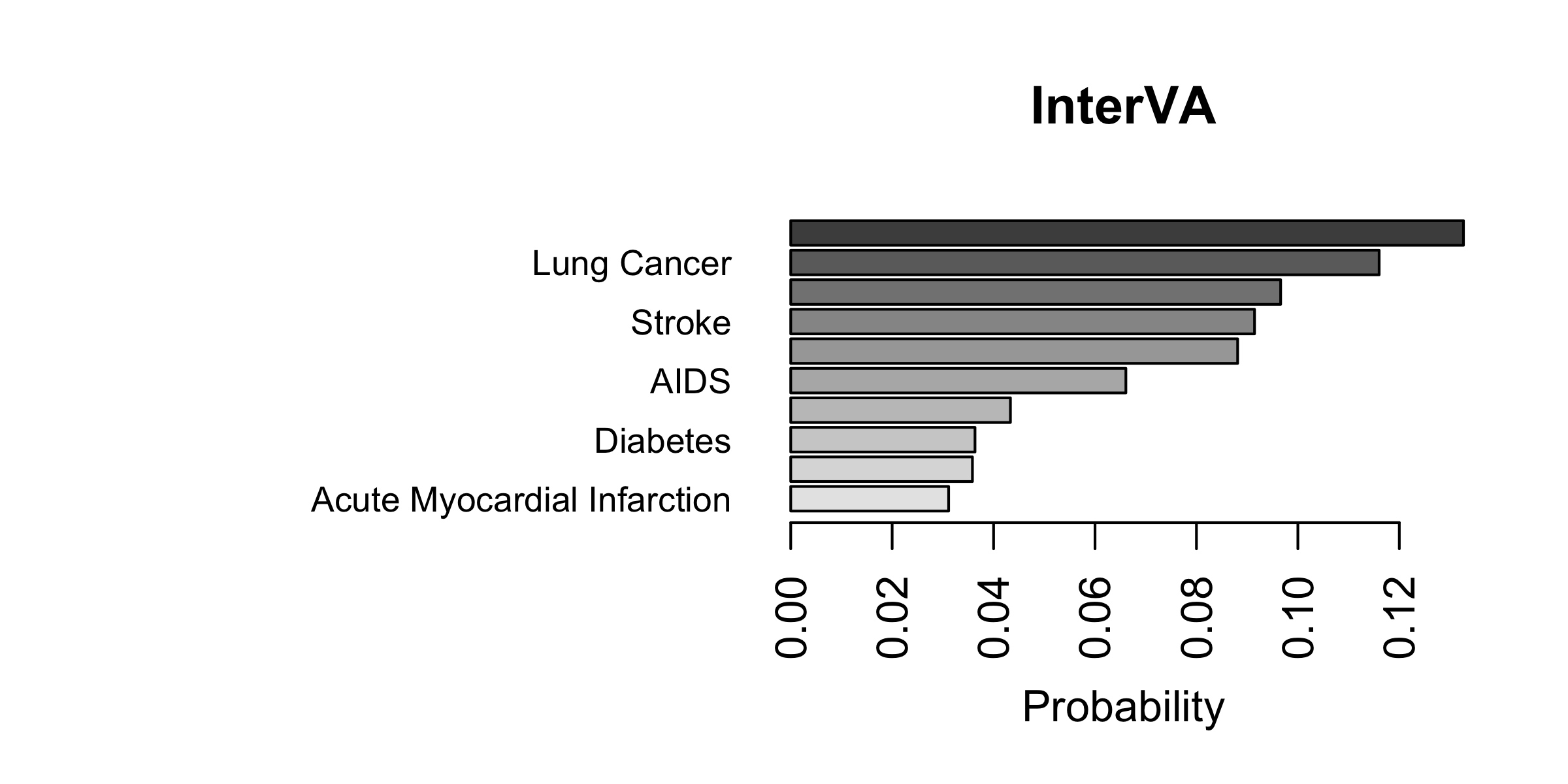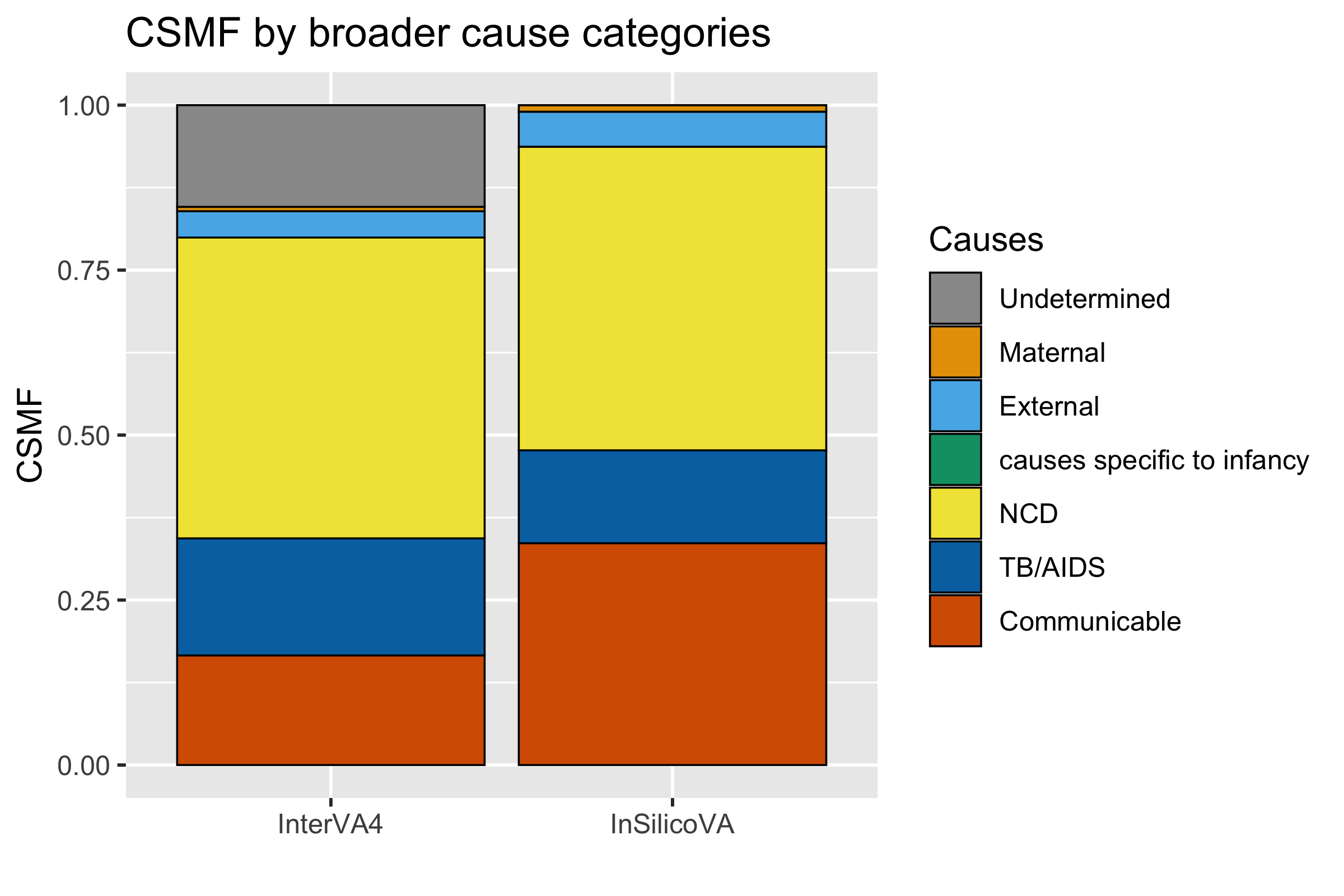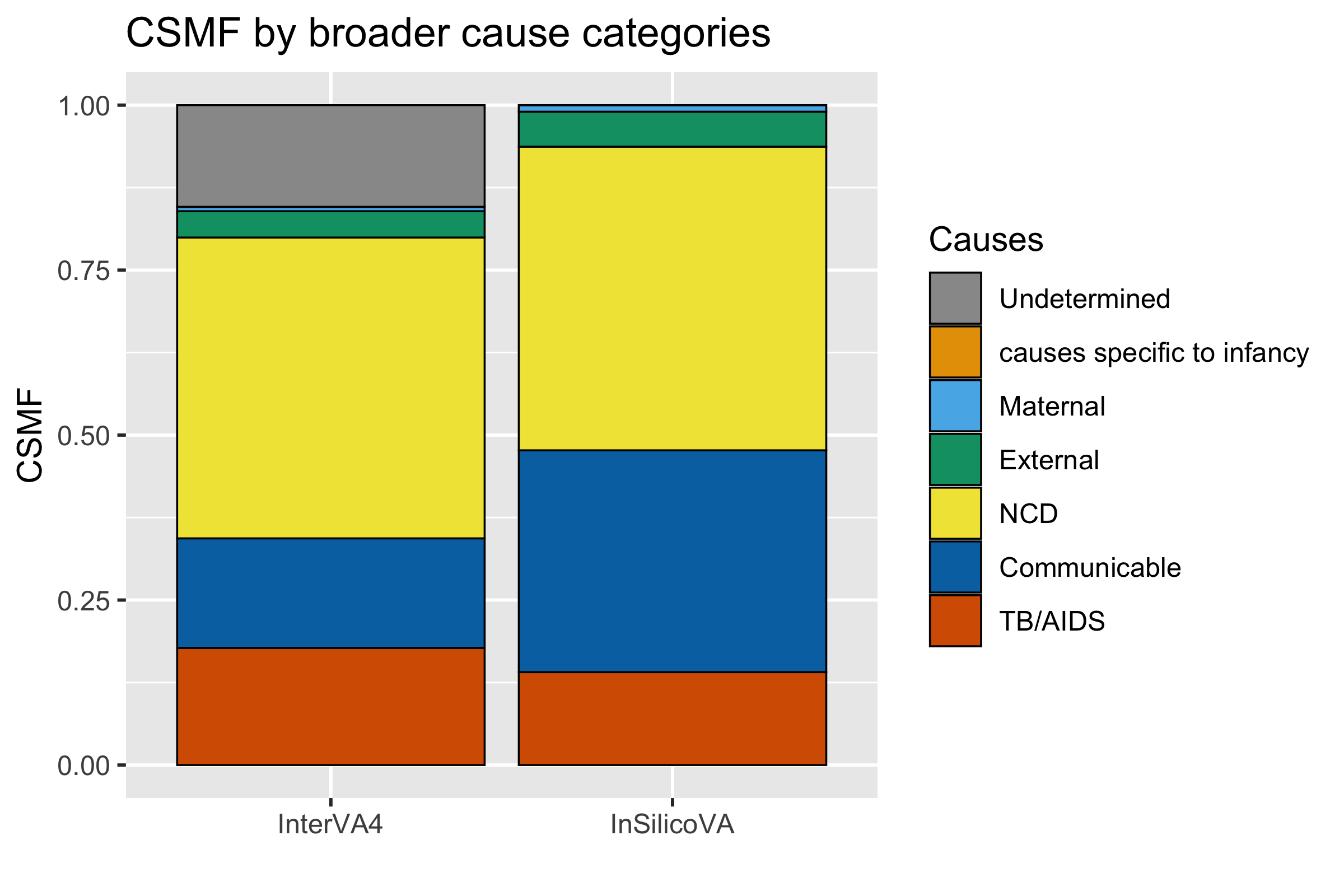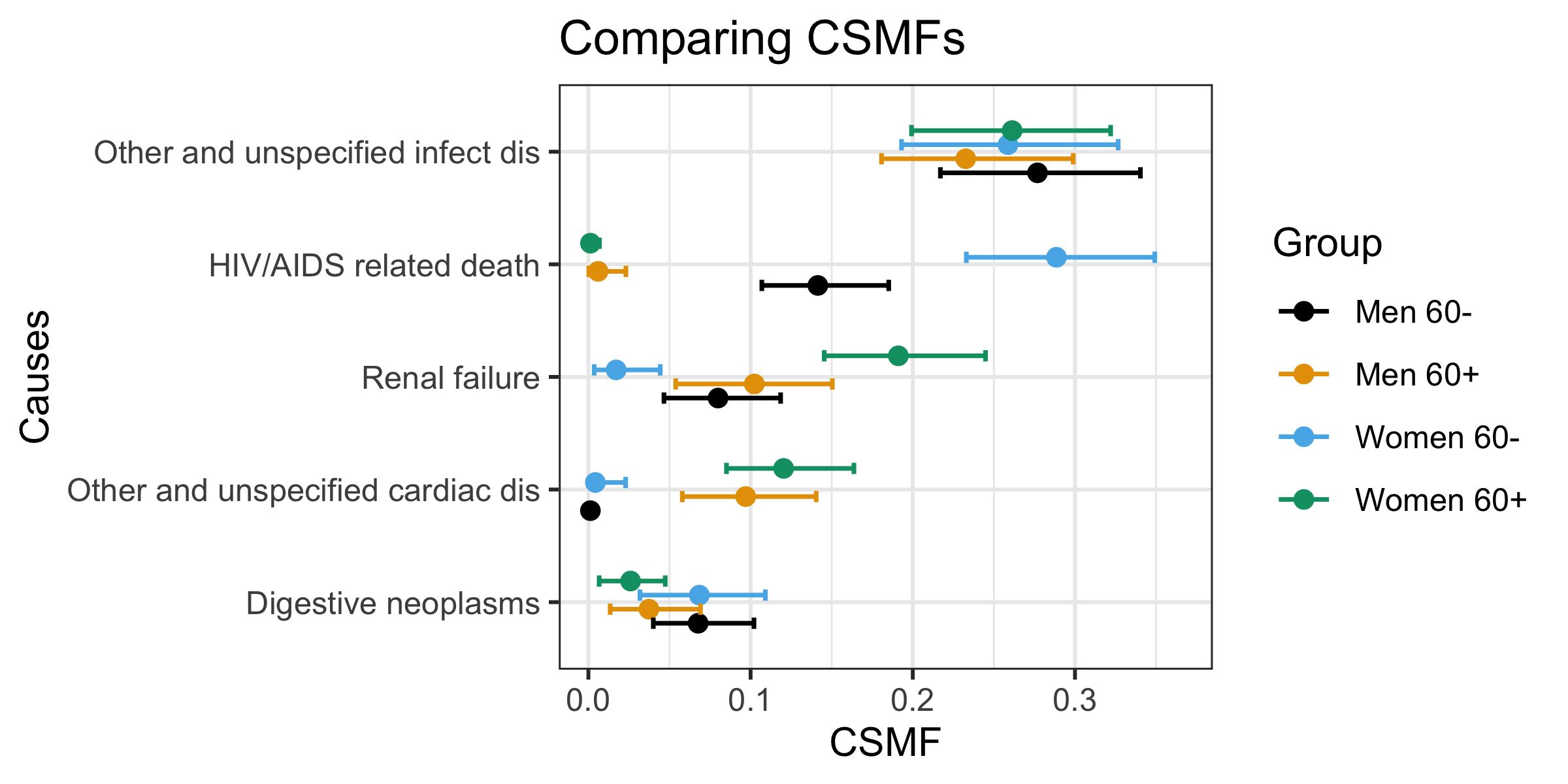# Introduction

Verbal autopsy (VA) is a well-established approach to ascertaining the cause of death (COD) when medical certification and full autopsies are not feasible or practical (Garenne 2014; Taylor et al. 1983). After a death is identified, a specially-trained fieldworker interviews the caregivers (usually family members) of the decedent. A typical VA interview includes a set of structured questions with categorical or quantitative responses and a narrative section that records the “story” of the death from the respondent’s point of view (World Health Organization 2012).

This vignette aims to provide a quick introduction to the openVA package using simple case studies. For more detailed discussion of the package and related VA methods, please refer to (Zehang R. Li et al. 2021)

## Overview of openVA

The openVA package (Zehang Richard Li et al. 2022) addresses this issue through an open-source toolkit. The openVA suite comprises a collection of R packages for the analysis of verbal autopsy data. The goal of this package is to provide researchers with an open-source tool that supports flexible data input formats and allows easier data manipulation and model fitting. The openVA suite consists of four core packages that are on CRAN, InterVA4 (Zehang R. Li et al. 2019), InterVA5 (Thomas et al. 2021), InSilicoVA (Zehang R. Li, McCormick, and Clark 2022), and Tariff (Zehang R. Li, McCormick, and Clark 2018), and an optional package nbc4va (Wen et al. 2018). Each of these packages implements one coding algorithm. For three of these algorithms – namely, InterVA-4, InterVA-5 and Tariff – there are also compiled software programs distributed by the original authors.

The openVA package is hosted on CRAN and can be installed with the following commands. For the analysis in this paper, we also install the nbc4va package separately from GitHub. The versions of the supporting packages can be checked in R using the openVA_status() function.

set.seed(12345)
library(openVA)
remotes::install_github("rrwen/nbc4va")
library(nbc4va)
openVA_status()

The common modeling framework for VA data consists of first converting the survey responses into a series of binary variables, and then assigning a COD to each death based on the binary input variables. Typically, the target of inference consists of two parts: the individual cause-of-death assignment, and the population-level cause-specific mortality fractions (CSMF), i.e., the fraction of deaths due to each cause. In this section, we formally compare the modeling approaches utilized by each algorithm for these two targets. We adopt the following notations. Consider $$N$$ deaths, each with $$S$$ binary indicators of symptoms. Let $$s_{ij}$$ denote the indicator for the presence of $$j$$-th symptom in the $$i$$-th death, which can take values 0, 1, or NA (for missing data). We consider a pre-defined set of causes of size $$C$$. For the $$i$$-th death, denote the COD by $$y_i \in \{1, ..., C\}$$ and the probability of dying from cause $$k$$ is denoted by $$P_{ik}$$. For the population, the CSMF of cause $$k$$ is denoted as $$\pi_k$$, with $$\sum_{k=1}^C \pi_k = 1$$.

• InterVA4 (Byass et al. 2012) and InterVA5 (Byass et al. 2019) algorithms calculate the probability of each COD given the observed symptoms using the following formula, $P_{ik} = \frac{\pi_{k}^{(0)} \prod_{j=1}^S P(s_{ij}=1|y_{i}=k) \mathbf{1}_{s_{ij} = 1}} {\sum_{k' = 1}^C \pi_{k'}^{(0)} \prod_{j=1}^S P(s_{ij}=1|y_{i}=k') \mathbf{1}_{s_{ij} = 1}}$ where both the prior distribution of each of the causes, $$\pi_{k}^{(0)}$$ and the conditional probabilities $$P(s_{ij} = 1 | y_i = k)$$ are fixed values provided in the InterVA software. It is worth noting that the formula does not follow the standard Bayes’ rule as it omits the probability that any symptom is absent. A detailed discussion of this modeling choice can be found in McCormick et al. (2016). The conditional probabilities, $$P(s_{ij}=1|y_{i}=k)$$, used in InterVA algorithms are represented as rankings with letter grades instead of numerical values (Byass et al. 2012). For example, $$P(s_{ij}=1|y_{i}=k) = A+$$ is translated into $$P(s_{ij}=1|y_{i}=k) = 0.8$$, etc. The standard InterVA software only supports the fixed set of symptoms and causes where such prior information is provided. For a different data input format, this formulation can be easily generalized if training data are available. We include in the openVA package an extension of the algorithm that calculates $$\hat P(s_{ij}=1|y_{i}=k)$$ from the empirical distribution in the training data and then maps to letter grades with different truncation rules. Details of the extended InterVA algorithm can be found in McCormick et al. (2016).

After the individual COD distributions are calculated, InterVA-4 utilizes a series of pre-defined rules to identify up to the top three most likely COD assignments, and truncates the probabilities for the rest of the CODs to 0 and adds an ‘undetermined’ category so that the probabilities sum up to 1 (See the user guide of Byass (2015)). Then the population-level CSMFs are calculated as the aggregation of individual COD distributions, such that $\pi_k = \sum_{i=1}^N P^*_{ik}$ where $$P^*_{ik}$$ denotes the individual COD distribution after introducing the undetermined category.

• Naive Bayes Classifier (Miasnikof et al. 2015) is very similar to the InterVA algorithm with two major differences. First, instead of considering only symptoms that present, the NBC algorithm also considers symptoms that are absent. Second, the conditional probabilities of symptoms given causes are calculated from training data instead of given by physicians, which is similar to our extension of InterVA discussed above. Similar to InterVA, the NBC method can be written as $P_{ik} = \frac{\pi_{k}^{(0)} \prod_{j=1}^S (P(s_{ij}=1|y_{i}=k) \mathbf{1}_{s_{ij} = 1} + P(s_{ij} \neq 1|y_{i}=k) \mathbf{1}_{s_{ij} \neq 1})} {\sum_{k' = 1}^C \pi_{k'}^{(0)} \prod_{j=1}^S (P(s_{ij}=1|y_{i}=k') \mathbf{1}_{s_{ij} = 1}+ P(s_{ij} \neq 1|y_{i}=k') \mathbf{1}_{s_{ij} \neq 1})}$ and the CSMFs are calculated by $$\pi_k = \sum_{i=1}^N P_{ik}$$.

• InSilicoVA algorithm (McCormick et al. 2016) assumes a generative model that characterizes both the CSMF at the population level, and the COD distributions at the individual level. In short, the core generative process assumes $\begin{eqnarray} s_{ij} | y_i = k &\propto& \mbox{Bernoulli}(P(s_{ij} | y_i = k)) \\ y_i | \pi_1, ..., \pi_C &\propto& \mbox{Categorical}(\pi_1, ..., \pi_C) \\ \pi_k &=& \exp \theta_k / \sum_{k=1}^C \exp \theta_k \\ \theta_k &\propto& \mbox{Normal}(\mu, \sigma^2) \end{eqnarray}$ and hyperpriors are also placed on $$P(s_{ij} | y_i = k)$$, $$\mu$$, and $$\sigma^2$$. The priors for $$P(s_{ij} | y_i = k)$$ are set by the rankings used in InterVA-4 if the data are prepared into InterVA format, otherwise they are learned from training data. The priors on $$\mu$$ and $$\sigma^2$$ are diffuse uniform priors. Parameter estimation is performed using MCMC, so that a sample of posterior distributions of $$\pi_k$$ can be obtained after the sampler converges.

• Tariff algorithm (James et al. 2011) differs from the other three methods in that it does not calculate an explicit probability distribution of the COD for each death. Instead, for each death $$i$$, a Tariff score is calculated for each COD $$k$$ so that $Score_{ik} = \sum_{j = 1}^{S} \mbox{Tariff}_{kj}\mathbf{1}_{s_{ij}=1}$ where the symptom-specific Tariff score $$\mbox{Tariff}_{kj}$$ is defined as $\mbox{Tariff}_{kj} = \frac{n_{kj} - median(n_{1j}, n_{2j}, ..., n_{Cj})} {IQR(n_{1j}, n_{2j}, ..., n_{Cj})}$ where $$n_{kj}$$ is the count of how many deaths from cause $$k$$ contain symptom $$j$$ in the training data. The Tariff scores are then turned into rankings by comparing them to a reference distribution of scores calculated from re-sampling the training dataset to obtain a uniform COD distribution. It is worth noting that the Tariff algorithm produces the COD distribution for each death in terms of their rankings instead of the probability distributions. And thus the CSMF for each cause $$k$$ is calculated by the fraction of deaths with cause $$k$$ being the highest ranked cause, i.e., $\pi_k = \frac{\sum_{i=1}^N\mathbf{1}_{y_i = k}}{N}$

In addition to the different model specifications underlying each algorithm, there is also a major conceptual difference in handling missing symptoms across the algorithms. Missing symptoms could arise from different stages of the data collection process. For example, the respondent may not know whether certain symptoms existed or may refuse to answer a question. From a statistical point of view, knowing that a symptom does not exist provides some information to the possible cause assignment, while a missing symptom does not. Although in theory, most of the VA algorithms could benefit from distinguishing ‘missing’ from ‘absent’, InSilicoVA is the only algorithm that has been implemented to acknowledge missing data. Missing indicators are assumed to be equivalent to ‘absence’ in InterVA, NBC, and Tariff.

# Data preparation

In the openVA package, we consider two main types of standardized questionnaire: the WHO instrument and the IHME questionnaire. In this section, we focus on describing these two data formats and tools to clean and convert data. Pre-processing the raw data collected from the survey instrument (usually with Open Data Toolkit) is usually performed with additional packages and software outside of the analysis pipeline in R. We briefly mention software for data pre-processing towards the end of this paper.

## The WHO standard format

For users familiar with InterVA software and the associated data processing steps, the standard input format from the WHO 2012 and 2016 instruments is usually well understood. For the 2012 instrument, the data expected by the InterVA-4 software are organized into a data frame where each row represents one death and the corresponding VA information is contained in $$246$$ fields, starting from the first item being the ID of the death. The $$245$$ items following the ID each represent one binary variable of symptom/indicator, where ‘presence’ is coded by ‘Y’, and ‘absence’ is coded by an empty cell.

To accommodate updates for the WHO 2016 instrument (D’Ambruoso et al. 2017), the InterVA-5 software accepts a data frame with $$354$$ columns that include $$353$$ columns of symptom/indicators followed by an additional column for the record ID. It should be noted that the R package InterVA5 retains the format with the record ID residing in the first column. Another important update with InterVA-5 is that it acknowledges the difference between “Yes” and “No” (or “Y/y” and “N/n”, which is different from the coding scheme in InterVA-4), both of which are processed as relevant responses, while all other responses are treated as missing values and ignored. With respect to the list of causes of death, InterVA-5 utilizes the WHO 2016 COD categories, which is nearly identical to the WHO 2012 COD categories (used by InterVA-4) except that hemorrhagic fever and dengue fever are two separate categories in the 2016 COD categories.

The same input format is inherited by the openVA package, except for one modification. We further distinguish ‘missing’ and ‘absent’ in the input data frame explicitly. We highly recommend that users pre-process all the raw data so that a ‘missing’ value in the data spreadsheet is coded as a ‘.’ (following the Stata practice familiar to many VA practitioners), and an ‘absent’ value is indicated by an empty cell, as in the standard InterVA-4 software. For WHO 2016 data, both ‘.’ and ‘-’ (following the default coding scheme of InterVA-5 software) are interpreted as missing values. For methods other than InSilicoVA, ‘missing’ and ‘absent’ will be considered the same internally and thus will not introduce a compatibility problem.

## The PHMRC format

The Population Health Metrics Research Consortium (PHMRC) gold standard VA data (Murray et al. 2011) consist of three datasets corresponding to adult, child, and neonatal deaths, respectively. All deaths occurred in health facilities and gold-standard causes are determined based on laboratory, pathology and medical imaging findings. These datasets can be downloaded directly using the link returned by the function getPHMRC_url(). For example, we can read the adult VA dataset using the following command.

PHMRC_adult <- read.csv(getPHMRC_url("adult"))

Although the data are publicly accessible, a major practical challenge for studies involving the PHMRC gold standard dataset is that the pre-processing steps described from the relevant publications are not clear enough nor easy to implement. The openVA package internally cleans up the PHMRC gold standard data when calling the codeVA() function on the PHMRC data. The procedure follows the steps described in the supplement material of McCormick et al. (2016). Notice that the original PHMRC data are useful for comparing and validating new methods, as well as for using as training data, but the cleaning functions only require that the columns are exactly the same as the PHMRC gold standard datasets, so they could also be used for new data that are pre-processed into the same format.

## Customized format

In addition to the two standard questionnaires discussed previously, researchers might also be interested in including customized dichotomous symptoms in their analysis. The openVA package also supports customized inputs as long as they are dichotomous. In such case, neither the built-in conditional probability matrix of InterVA nor the PHMRC gold standard dataset could be used to learn the relationship between training and testing data, thus different training data with known causes of death are necessary for all three algorithms. The ConvertData() function can be used to convert data with customized coding schemes into the format recognized by the openVA package.

# Modeling data collected with WHO 2012 questionnaire

In the first example, we demonstrate fitting InterVA and InSilicoVA on a random sample of $$1,000$$ deaths from the ALPHA network without cause-of-death labels collected with the WHO 2012 instrument. The dataset is already included in the openVA package as a dataset RandomVA1 and can be loaded directly.

data(RandomVA1)
dim(RandomVA1)
##  1000  246
head(RandomVA1[, 1:10])
##   ID elder midage adult child under5 infant neonate male female
## 1 d1     Y                                             Y
## 2 d2     Y                                                    Y
## 3 d3            Y                                      Y
## 4 d4                  Y                                       Y
## 5 d5                  Y                                Y
## 6 d6                  Y                                       Y

The codeVA() function provides a standardized syntax to fit different VA models. Internally, the codeVA() function organizes the input data according to the specified data type, checks for incompatibility of the data and specified model, and calls the corresponding model fitting functions. It returns a classed object of the specified model class. In this example, we use version 4.03 of the InterVA software, which is the latest release of the original software compatible with the WHO 2012 instrument. Any additional model-specific parameters can be passed through the arguments of codeVA(). Here we specify the HIV and malaria prevalence levels required by the InterVA model to be ‘high’. Guidelines on how to set these parameters can be found in Byass et al. (2012).

fit_inter_who <- codeVA(data = RandomVA1, data.type = "WHO2012",
model = "InterVA", version = "4.03",
HIV = "h", Malaria = "h")
summary(fit_inter_who) 
## InterVA-4 fitted on 1000 deaths
## CSMF calculated using reported causes by InterVA-4 only
## The remaining probabilities are assigned to 'Undetermined'
##
## Top 5 CSMFs:
##  cause                     likelihood
##  Undetermined              0.154
##  HIV/AIDS related death    0.122
##  Stroke                    0.072
##  Reproductive neoplasms MF 0.058
##  Pulmonary tuberculosis    0.055

We can implement InSilicoVA method with similar syntax. We use the default parameters and run the MCMC for $$10,000$$ iterations. Setting the auto.length argument to FALSE specifies that the algorithm does not automatically increase the length of the chain when convergence failed. The InSilicoVA algorithm is implemented using a Metropolis-Hastings within Gibbs sampler. The acceptance rate is printed as part of the message as the model samples from the posterior distribution.

fit_ins_who <- codeVA(RandomVA1, data.type = "WHO2012", model = "InSilicoVA",
Nsim = 10000, auto.length = FALSE)
summary(fit_ins_who) 
## InSilicoVA Call:
## 1000 death processed
## 10000 iterations performed, with first 5000 iterations discarded
##  250 iterations saved after thinning
## Fitted with re-estimated conditional probability level table
## Data consistency check performed as in InterVA4
##
## Top 10 CSMFs:
##                                    Mean Std.Error Lower Median Upper
## Other and unspecified infect dis  0.266    0.0168 0.235  0.265 0.301
## HIV/AIDS related death            0.102    0.0091 0.085  0.102 0.119
## Renal failure                     0.101    0.0108 0.084  0.101 0.123
## Other and unspecified neoplasms   0.062    0.0089 0.046  0.061 0.080
## Other and unspecified cardiac dis 0.058    0.0076 0.044  0.058 0.075
## Digestive neoplasms               0.050    0.0077 0.033  0.050 0.065
## Acute resp infect incl pneumonia  0.048    0.0073 0.034  0.049 0.063
## Pulmonary tuberculosis            0.039    0.0068 0.025  0.039 0.054
## Stroke                            0.038    0.0061 0.027  0.038 0.052
## Other and unspecified NCD         0.034    0.0089 0.018  0.034 0.052

# Modeling the PHMRC data

In the second example, we consider a prediction task using the PHMRC adult dataset. We first load the complete PHMRC adult dataset from its on-line repository, and organize it into training and test datasets. We treat all deaths from Andhra Pradesh, India as the test dataset.

PHMRC_adult <- read.csv(getPHMRC_url("adult"))

## Individual COD summary

At the individual level, we can extract the most likely cause-of-death assignment from the fitted object using the getTopCOD() function.

cod_inter <- getTopCOD(fit_inter)
cod_ins <- getTopCOD(fit_ins)
cod_nbc <- getTopCOD(fit_nbc)
cod_tariff <- getTopCOD(fit_tariff)

With the most likely COD assignment, other types of metrics based on individual COD assignment accuracy can be similarly constructed by users. The summary methods can also be called for each death ID. For example, using the Tariff method, we can extract the fitted rankings of causes for the death with ID 6288 by

summary(fit_inter, id = "6288")
## InterVA-4 fitted top 5 causes for death ID: 6288
##
##  Cause                     Likelihood
##  Stroke                    0.509
##  Pneumonia                 0.318
##  COPD                      0.081
##  Other Infectious Diseases 0.064
##  Renal Failure             0.013

The summary() function for InSilcoVA does not provide uncertainty estimates for individual COD assignments by default. This is because, in practice, the calculation of individual posterior probabilities of COD distribution can be memory-intensive when the dataset is large. To obtain individual-level uncertainty measurements, we can either run the MCMC chain with the additional argument indiv.CI = 0.95 when calling codeVA(), or update the fitted object directly with the saved posterior draws.

fit_ins <- updateIndiv(fit_ins, CI = 0.95)
summary(fit_ins, id = "6288")
## InSilicoVA fitted top  causes for death ID: 6288
## Credible intervals shown: 95%
##                               Mean  Lower Median  Upper
## Stroke                      0.5043 0.3485 0.5083 0.6361
## Pneumonia                   0.4116 0.2615 0.4083 0.5834
## Other Infectious Diseases   0.0660 0.0411 0.0642 0.0966
## Epilepsy                    0.0099 0.0064 0.0097 0.0142
## COPD                        0.0053 0.0031 0.0052 0.0079
## Malaria                     0.0007 0.0005 0.0007 0.0011
## Diabetes                    0.0005 0.0003 0.0005 0.0009
## Acute Myocardial Infarction 0.0004 0.0003 0.0004 0.0006
## Falls                       0.0004 0.0001 0.0004 0.0013
## Renal Failure               0.0004 0.0002 0.0003 0.0005

For $$N$$ deaths, $$C$$ causes, the posterior mean of individual COD distributions returned by the InSilicoVA model, along with median and with credible intervals can be represented by a $$(N \times C \times 4)$$-dimensional array. The function getIndivProb() extracts this summary in the form of a list of $$4$$ matrices of dimension $$N$$ by $$C$$, which can then be saved to other formats to facilitate further analysis. For other methods, the point estimates of individual COD distribution are returned as the $$N$$ by $$C$$ matrix.

fit_prob <- getIndivProb(fit_inter)
dim(fit_prob)
##  1554   34

## Visualization

The previous sections discuss how results could be extracted and examined in R. In this subsection, we show some visualization tools provided in the openVA package for presenting these results. The fitted CSMFs for the top causes can be easily visualized by the plotVA() function. The default graph components are specific to each algorithm and individual package implementations, with options for further customization. For example, the figure below shows the estimated CSMF from the InterVA algorithm in the PHMRC data example.

plotVA(fit_inter, title = "InterVA")Top 10 CSMFs estimated by InterVA.

The CSMFs can also be aggregated for easier visualization of groups of causes. For the InterVA-4 cause list, we included an example grouping built into the package, so the aggregated CSMFs can be compared directly. In practice, the grouping of causes of deaths often needs to be determined according to context and the research question of interest. Changing the grouping can be easily achieved by modifying the grouping argument in the stackplotVA() function. For example, to facilitate the new category of Undetermined returned by InterVA, we first modify the grouping matrix to include it as a new cause and visualize the aggregated CSMF estimates in the figure below.

data(SampleCategory)
grouping <- SampleCategory
grouping[,1] <- as.character(grouping[,1])
grouping <- rbind(grouping, c("Undetermined", "Undetermined"))
compare <- list(InterVA4 = fit_inter_who,
InSilicoVA = fit_ins_who)
stackplotVA(compare, xlab = "", angle = 0,  grouping = grouping)Estimated aggregated CSMFs for InterVA-4 and InSilicoVA, adding undetermined category.

The ordering of the stacked bars can also be changed to reflect the structures within the aggregated causes, as demonstrated in the figure below.

group_order <- c("TB/AIDS",  "Communicable", "NCD", "External", "Maternal",
"causes specific to infancy", "Undetermined")
stackplotVA(compare, xlab = "", angle = 0, grouping = grouping,
group_order = group_order)Estimated aggregated CSMFs for InterVA-4 and InSilicoVA, with the causes reordered.

# Incorporating additional information in the InSilicoVA algorithm

Among the VA methods discussed in this paper, the InSilicoVA algorithm (McCormick et al. 2016) allows for more flexible modifications to the Bayesian hierarchical model structure when additional information is available. In this section, we illustrate two features unique to the InSilicoVA method: jointly estimating CSMFs from multiple populations, and incorporating partial and potentially noisy physician codings into the algorithm.

## Sub-population specific CSMFs

In practice researchers may want to estimate and compare CSMFs for different regions, time periods, or demographic groups in the population. Running separate models on subsets of data can be inefficient and does not allow parameter estimation to borrow information across different groups. The generative framework adopted by InSilicoVA allows the specification of sub-populations in analyzing VA data. Consider an input dataset with $$G$$ different sub-populations. We can estimate different CSMFs $$\pi^{(g)}$$ for $$g = 1, ..., G$$ for each sub-population, while assuming the same conditional probability matrix, $$P_{s|c}$$ and other hyperpriors. As an example, we show how to estimate different CSMFs for sub-populations specified by sex and age groups, using a randomly sampled ALPHA dataset with additional columns specifying the sub-population each death belongs to.

data(RandomVA2)
head(RandomVA2[, 244:248])
##   stradm smobph scosts   sex age
## 1      .      .      .   Men 60+
## 2      .      .      . Women 60-
## 3      .      .      . Women 60-
## 4      .      .      . Women 60+
## 5      .      .      . Women 60-
## 6      .      .      . Women 60-

Then we can fit the model with one or multiple additional columns specifying sub-population membership for each observation.

fit_sub <- codeVA(RandomVA2, model = "InSilicoVA",
subpop = list("sex", "age"),  indiv.CI = 0.95,
Nsim = 10000, auto.length = FALSE)

Functions discussed in the previous sections work in the same way for the fitted object with multiple sub-populations. Additional visualization tools are also available. The figure below plots the CSMFs for two sub-populations on the same plot by specify type = "compare".

plotVA(fit_sub, type = "compare", title = "Comparing CSMFs", top = 3)Estimated CSMFs for different sub-populations. The points indicate posterior means of the CSMF and the error bars indicate 95% credible intervals of the CSMF.

By default, the comparison plots will select all the CODs that are included in the top $$K$$ for each of the sub-populations. We can also plot only subsets of them by specifying the causes of interest, as shown in the figure below.

plotVA(fit_sub, type = "compare", title = "Comparing CSMFs",
causelist = c("HIV/AIDS related death",
"Pulmonary tuberculosis",
"Other and unspecified infect dis",
"Other and unspecified NCD"))